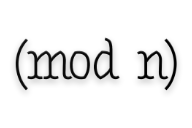# mod – modular arithmetic in Python¶## Description¶

Modular arithmetic is arithmetic for integers, where numbers wrap around when reaching a given value called modulus. For example 6 ≡ 1 (mod 5). Modular arithmetic has several practical applications including: music, banking, book publishing, cryptography… and of course math.

The purpose of this package is to simplify the use of modular arithmetic in Python3.## Usage¶

This package provides Mod integers that compute arithmetic operations like + - * // ** with a modulus:

from mod import Mod

# Funny math here

x = Mod(5, 7)      # x ≡ 5 (mod 7)

(x + 2) == 0       # True: 5 + 2 ≡ 7 ≡ 0 (mod 7)
(x + 7) == x       # True: 5 + 7 ≡ 12 ≡ 5 (mod 7)
(x**3) == (x + 1)  # True: 5³ ≡ 125 ≡ 6 (mod 7)
(1 // x) == 3      # True: 5 × 3 ≡ 15 ≡ 1 (mod 7) ⇒ 5⁻¹ ≡ 3 (mod 7)


A naive implementation of RSA encryption algorithm using mod package:

from mod import Mod

# My RSA keys
public_key = Mod(3, 61423)
private_key = Mod(40619, 61423)

# My very secret message
top_secret_message = 666

# RSA encryption
encrypted = top_secret_message**public_key

# RSA decryption
decrypted = encrypted**private_key

# My secret message have been correctly encrypted and decrypted :-)
assert decrypted == top_secret_message


Note

• Mod is based on integer modulo operation %, not math.fmod
• the result of an operation between a Mod and an int is a Mod
• the result of an operation between a Mod and a float is a float

## Package documentation: mod.Mod¶

class mod.Mod(value, modulus)

Integer number that automatically adds a modulus to arithmetic operations.

The floor division // implements the inverse of a multiplication with a modulus. Therefore, it should be used with care to avoid errors.

>>> number = Mod(2, 3)
>>> number
(2 % 3)
>>> quintuple = 5 * number
>>> quintuple // 5
(2 % 3)
>>>

Parameters: value (int) – Mod number value modulus (int) – modulus associated with the value ValueError – one of the parameters is not a number or modulus == 0
copy(modulus=None)

Copy the Mod number

Parameters: modulus (int) – modulus of the new Mod number Mod
inverse

Modular inverse of the number.

y is the inverse of x with the modulus n when:

$y × x ≡ 1 (mod. n)$
Return type: Mod
modulus

Modulus value

Return type: int

## Install¶

Run the following command to install mod package

pip3 install mod